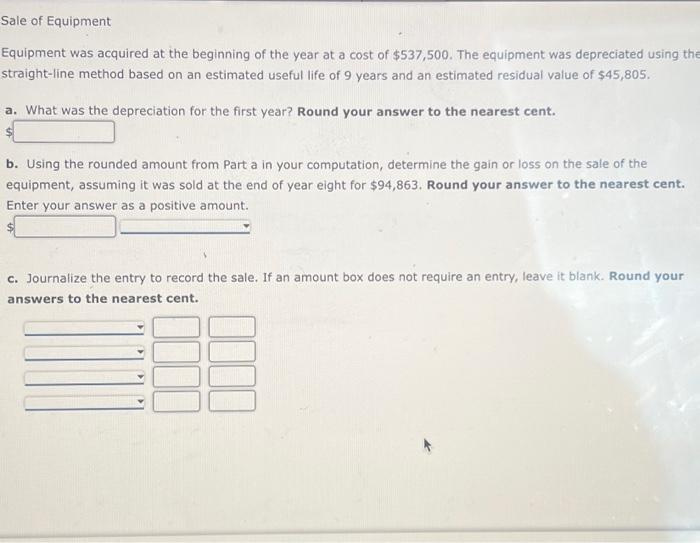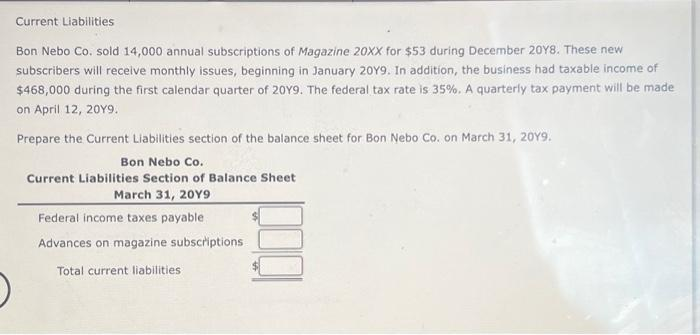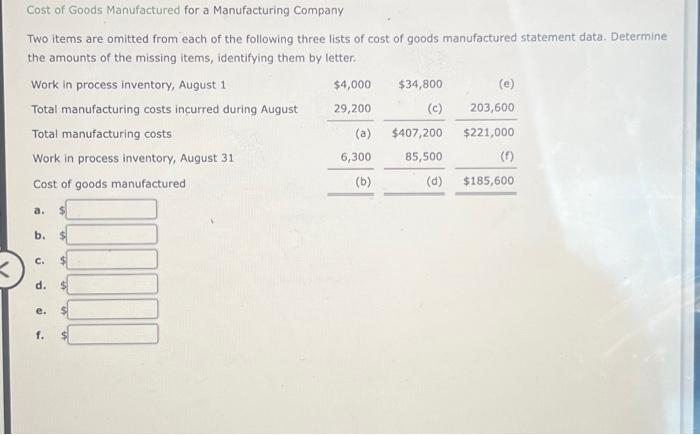# (Solved): all parts or dont answer thank you b. Using the rounded amount from Part a in your computation, det ...

all parts or dont answer thank youWe have an Answer from Expert

Sol:-
1)a) Calculation of Depreciation for the first year:-

Therefore, Depreciation for the first year = \$107,500

Depreciation for the first year = Beginning Year Equipment Cost x Double - Declining Method Percentage

Working Note:-

Useful life = 10 years
Double declining depreciation rate = 2 x 1/10
Double declining depreciation rate = 20%

Double declining depreciation rate = 2 x 1/useful life

We have an Answer from Expert